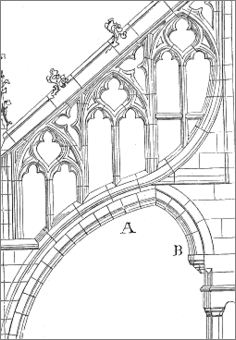# Drawing an arc in Canvas: Tutorial and interactive demo

The arc is the basis for many geometric figures, it has a symmetrical shape and may be defined from two angles.Simple plot, elaborate uses

A combination of arcs can form such a figure as that on the right where the orientation may vary but in which each curve fragment is an arc and therefore is symmetric.
To achieve non-symmetrical figures, curves functions will be used instead.

## The arc method depends on a position, a radius and two angles

The arc function has six parameters:

1. The starting point horizontally.
2. The vertical starting point.
4. The starting angle. Determines the initial inclination.
5. The ending angle. Determines the curvature.
6. A flag to reverse the direction of rotation: false sense of clockwise, true otherwise.

This gives the following syntax:

``arc(x, y, radius, startingangle, endingangle,  [true | false])``

Example of arc instruction:

``context.arc(100,5, 70, Math.PI, 1,5 * Math.PI,  true); ``

The point x, y can be the beginning of a new figure, in this case the beginPath method is called befor arc(). The arc can also be seen as an extension of a figure already drawn, then it retrieves the attributes of it and the position of the last drawn dot is its starting position.

A simple demo...

The starting angle is 0 and the final angle is PI. The radius is 70 pixels. The rotation direction is false (clock-wise):

The code:

``context.arc(150, 5, 70, 0, Math.PI, false); ``

Changing the direction of rotation

By default the rotation is clock-watch and it is false. We can reverse the direction with an optional sixth parameter.

If we give a true sixth parameter, the arc will be drawn in the opposite direction, counterclockwise.
Example as above but with a direction of rotation reversed:

Code:

`` context.arc(150, 75, 70, 0, Math.PI, true);  ``

## How to define angles of the arc

The angle has a real value ranging from 0 to 2 times PI, that is expressed in JavaScript by 2 * Math.PI.
The latter value corresponds to 360 ° (but the angle values ​​are not expressed in degrees).
The above example with initial angle of PI and final double PI:

Code:

``context.arc(150, 75, 70, Math.PI, 2 * Math.PI, false);``

And we face the previous arc as we have in each case the complement of the angle of the first example.

Making a arc to the left

When we trace in the direction of the clock, a semicircle has a starting of 0 and a ending angle of PI.
A circle is 0 to 2 times PI.
To draw a semicircle on the left, so we start to PI / 2 and ends at 1.5 times PI. Proof:

To draw an arc on the right, is assigned the value true to the parameter in the direction of rotation or we take the values ​​of angles of 1.5 IP and 0.5 IP.

Arc in motion

To see how this works, you go into an animation to vary the starting angle and the final angle from 0 to 2*PI.

Value of initial and final angle:

Code of the demonstration:

``````var angle = 0;var delay;
function drawAngle(ctxa){  ctxa.arc(150, 50, 40, angle, angle);  ctxa.stroke();  angle+=0.1;
if(angle > 2 * Math.PI) { clearInterval(delay);return;}  document.getElementById("angle").value = Math.round(angle * 100)/100;}
function setAngle(){  angle=0;  var canvas = document.getElementById("canvas4");   var ctxa = canvas.getContext("2d");  ctxa.lineWidth="3";
ctxa.beginPath();  delay = setInterval(function(){ drawAngle(ctxa); }, 100);}
setAngle();  ``````

## Interactive demonstration

How to choose a starting and ending angle, how to choose a sense of rotation, and the interaction between all these factors...
The best way to understand them is an interactive demonstration .

The Canvas tag
X max: 400
Y max: 200
Angle max: 2 PI = 6,29
Canvas requires a modern browser: Chrome, Firefox, IE9, Safari.
Defining an arc

Starting angle Ending angle Rotating direction: true false

Coordinates x= y= Radius (0-100)

The HTML code:

``````<canvas id="canvasDemo" width="402" height="202" style="border:1px solid #CCC;background:white">  Canvas requires a modern browser: Chrome, Firefox, IE9, Safari.
</canvas>``````

The JavaScript code:

``````function setArcDemo(){  var canvas = document.getElementById("canvasDemo");   var context = canvas.getContext("2d");    var x = document.getElementById("x").value;  var y = document.getElementById("y").value;  var radius = document.getElementById("radius").value;  var initial = document.getElementById("startangle").value;  var final = document.getElementById("endangle").value;  if(document.getElementById("clock1").checked)  	clock = true;  else	  	clock = false;  context.beginPath();  context.lineWidth="2";  context.arc(x, y, radius, initial, final, clock)  context.stroke();}
function erase(){  var canvas = document.getElementById("canvasDemo");   var context = canvas.getContext("2d");  context.clearRect(0,0, canvas.width, canvas.height); }``````

Next chapter: The arcTo method defines an arc from coordinates rather than an angle.